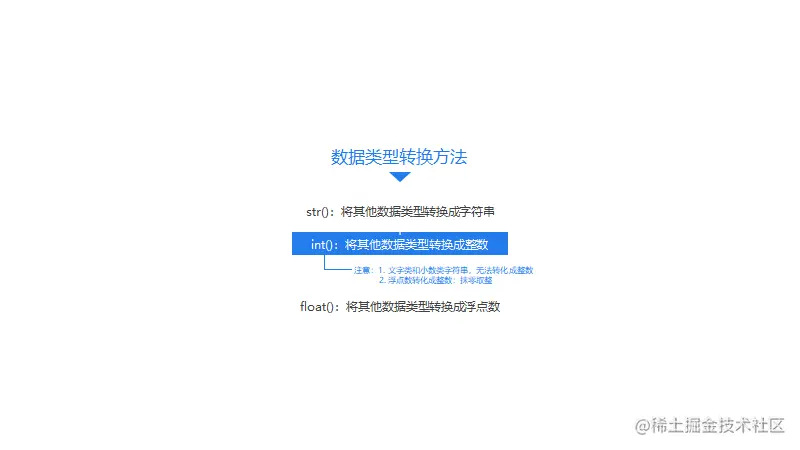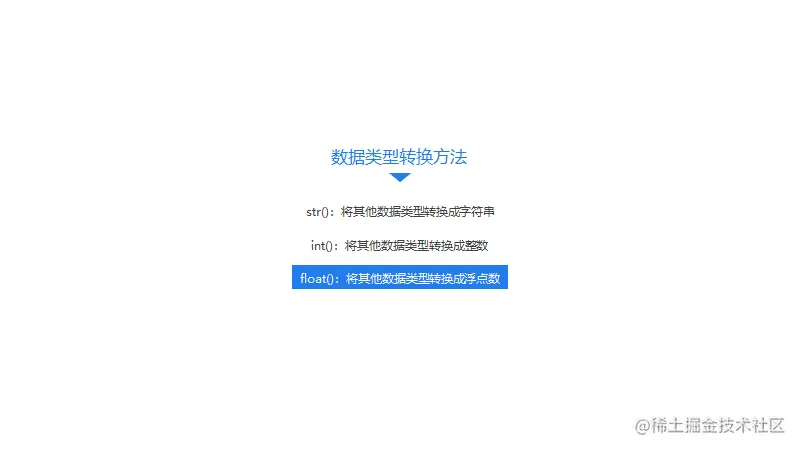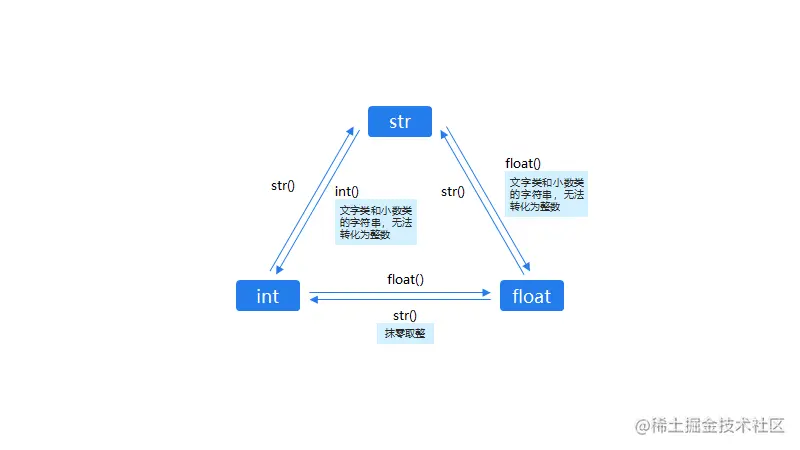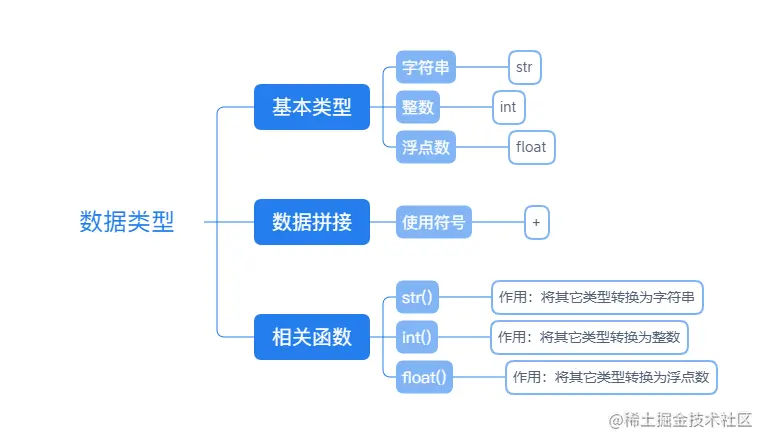# Python from 0 to 1 (day 13) - Python data application 3

2022-01-31 22:48:34

This is my participation 11 The fourth of the yuegengwen challenge 18 God , Check out the activity details ：2021 One last more challenge

### `int()` functionThe way to convert data to integer type is also very simple , Namely int() function . Its use method is the same as str() equally , Put the content you need to convert in parentheses , like this ：int( Converted content ).

Now let's try , To calculate the number1 and number2 The sum of these two variables .【 The point tip ：1. int() Data type conversion function ;2. Operator + Calculation ;3. print() function 】

``````number1 = '618'

number2 = '1111'

print(int(number1) + int(number2))
Copy code ``````

But for the `int() ` Use of functions , We should pay attention to ： Only those that conform to the integer specification String class data , Can be `int()` Coercive transformation .

Although it has only one sentence , But it has three meanings ： First , A string of integers, for example '618' and '1111', Can be `int() ` Function cast .

Last , String in decimal form , because Python Grammatical rules , Can't be used `int() ` Function cast .

Let's say the following code , If you click Run , The program will report an error 【ValueError：invalid literal for int() with base 10: '6.18'】（ Value anomaly ： Floating point type string cannot be used int() Coercive transformation ）.

``````print(int('6.18'))

#  Display the results after running ：ValueError：invalid literal for int() with base 10: '6.18'
Copy code ``````

Does that mean , Floating point number cannot be converted to integer type ？

No , Although floating-point form character string , Out of commission `int() ` function . but Floating point numbers Yes, it can be. `int() ` Function cast .

``````print(int(6.18))
Copy code ``````

The code string above , Is a floating point number 6 .18 Cast statement . however , Why the output is 6 Well ？

You see ,`int() ` The essence of a function is to convert data to integers . So for floating point numbers ,`int() ` The function does rounding . however , It is different from our usual method of rounding decimals ,`int() ` function Will erase directly , Direct output integer part .

Last , Let's summarize `int()` Knowledge points of function .But , If this happens ： A string is in decimal form , such as '3.8' such . I don't want it to be an integer 3, Want to keep it in decimal form 3.8, What am I going to do ？

This involves our last knowledge point ——`float()` function .

### `float()` functionFirst `float() ` Use of functions , Also put the data to be converted in brackets , like this ：`float( data )`.

secondly ,`float() ` The function can also put Integers and character string Convert to floating point type . But at the same time , If the data in brackets is character string type , Then the data must be in digital form .

that , After before `str() ` and `int() ` practice ,`float() ` Function is not easy to understand some ？

below , Let's play a piece of code and practice .

``````width = 40.0
length = 120.0
weight = "20"
print(width)
print(length)
print(float(weight))
Copy code ``````

In the last question ,`widht` And `lenght` The variable is already a floating point type ,`weight` It's a string type （ It can be used `type( Variable )` To see the data types ）, Therefore, before printing, it will `weight` Conduct `float(weight)` Just convert . Up to now , You already know how to convert data types . below , Let's summarize these three functions through a graph .What about? ？ Master the data type 、 You have three knowledge points of data splicing and data conversion , Do you have a sense of achievement to be a big man ？

that , Let's take a look at what you have learned ！But these , It's just the beginning , Later you will learn more complex code and commands , Master more communication skills with computers .